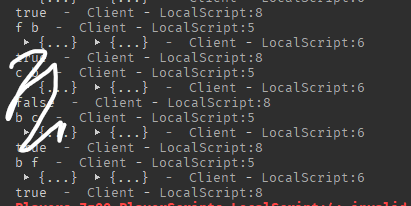# Table.sort error does not make sense?

``````local table_1 = {'a', 'b', 'c', 'd', 'e', 'f', 'g'}
local table_2 = {2, 4, 6, 5, 1, 4, 2}

table.sort(table_1, function(a, b)
return table_2[table.find(table_1, a)] > table_2[table.find(table_1, b)]
end)

print(table_1)
``````

`Error: invalid order function for sorting`

I was coding something similar to this code, I ran into a problem, I was confused why it errored so I tried to reproduce this error, I created the code above which always produce the error, does anyone know why it errors?

1 Like

I’m guessing it starts to go wrong at the f and b comparison.table.sort expects the comparison function to return a boolean such that if comparing a > b returns true, that will also mean that a cannot be greater than or equal to b (or that passing the elements in the opposite order must be false). In this case, comparing a > b (or in this case comparing f > b) will return true, but comparing b > f also returns true, as you can observe in the attached screenshot.

It’s a bit hard to explain, but I’ll try my best. Take for example the following:

``````local t = { 5; 4; 3; 2; 1; 1; 2; 3; 4; 5; }

table.sort(t, function(a, b)
return a >= b -- at first glance this appears to be the same as doing a < b, but what happens when we get to the point of comparing two equal numbers (say 5 and 5)?
-- comparing a >= b will give the same result as swapping the two arguments, which can't happen.
end)

-- now if we change our operator to a > b then,
table.sort(t, function(a, b)
return a > b -- 5 > 5 will return false. 5 > 4 for example will return true, however 4 <= 5 will return false therefore this sort function is valid
end)
``````